# Discovering Geometry Chapter 4: Discovering and Proving Triangle Properties Chapter Exam

Exam Instructions:

Choose your answers to the questions and click 'Next' to see the next set of questions. You can skip questions if you would like and come back to them later with the yellow "Go To First Skipped Question" button. When you have completed the practice exam, a green submit button will appear. Click it to see your results. Good luck!

### Page 1

#### Question 3 3. Which theorem or postulate can be used to establish congruence with the pictured triangles?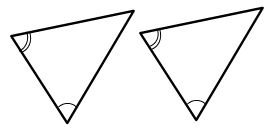#### Question 4 4. If triangle MNO is congruent to triangle PQR, CPCTC explains which of the following statements?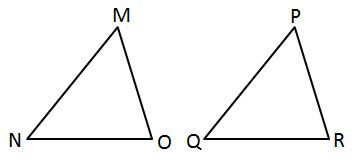#### Question 5 5. If angle a is 30 degrees and angle d is 70 degrees, what is the measure of angle b?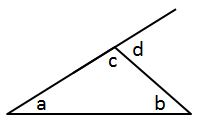### Page 2

#### Question 7 7. If angle a is 80 degrees, what is the measure of angle d?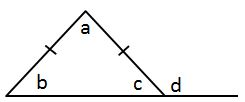#### Question 8 8. In the pictured triangle, AD is an angle bisector. What is the length of AC?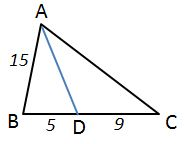#### Question 10 10. In the pictured triangles, what reason can we use to explain that angle QPR is congruent to angle SPT?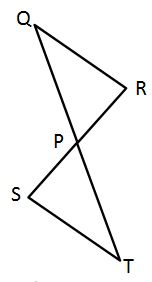### Page 3

#### Question 11 11. Which statement about the pictured triangle must be true?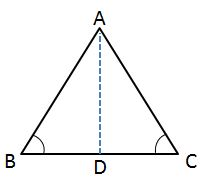#### Question 12 12. If angle a is 68 degrees and angle b is 37 degrees, what is the measure of angle c?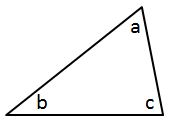#### Question 13 13. Which theorem or postulate can be used to establish congruence with the pictured triangles?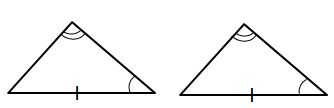#### Question 14 14. In the pictured triangle, FE is an angle bisector. What is the length of GE?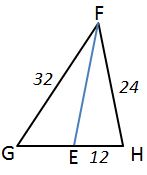#### Question 15 15. If angle a is 66 degrees and angle b is 54 degrees, what is the measure of angle d?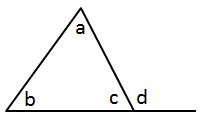### Page 4

#### Question 16 16. In the pictured triangle, MS is an angle bisector. What is the length of SO?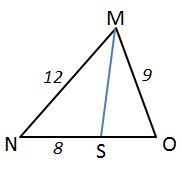#### Question 17 17. If the pictured triangles are congruent, what reason can be given?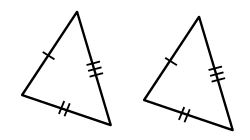#### Question 18 18. If the pictured triangles are congruent, what reason can be given?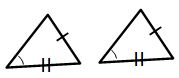#### Question 20 20. Which of the following represents the angle bisector theorem for the pictured triangle?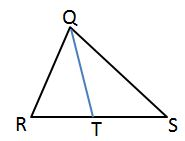### Page 5

#### Question 21 21. In the pictured triangle, XV is an angle bisector. What is the length of XY?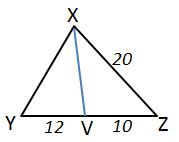#### Question 22 22. If triangle ABD is congruent to triangle CDB, CPCTC explains which of the following statements?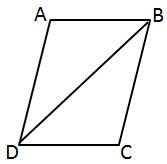#### Question 24 24. If angle a is 60 degrees, what is the measure of angle b?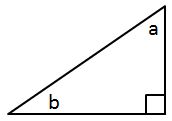#### Question 25 25. Which theorem or postulate can be used to establish congruence with the pictured triangles?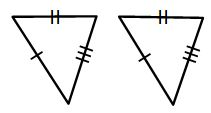### Page 6

#### Question 29 29. All of the following statements about the pictured triangle must be true, EXCEPT: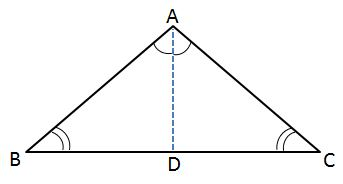#### Question 30 30. If the pictured triangles are congruent, what reason can be given?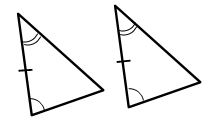#### Discovering Geometry Chapter 4: Discovering and Proving Triangle Properties Chapter Exam Instructions

Choose your answers to the questions and click 'Next' to see the next set of questions. You can skip questions if you would like and come back to them later with the yellow "Go To First Skipped Question" button. When you have completed the practice exam, a green submit button will appear. Click it to see your results. Good luck!

Support